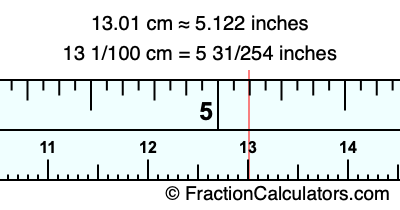13.01 cm in inchesHere is how to convert 13.01 cm to inches. We will give you the fractional answer, the decimal answer, and illustrate the answer on our tape measure.

One centimeter is equal to 50/127 inches, and 13.01 as a fraction is 13 1/100. Therefore, to get the fractional answer to 13.01 cm in inches, we multiply 13 1/100 by 50/127 and simplify if necessary.

cm × 50/127 = inches
13 1/100 × 50/127 = inches
13 1/100 × 50/127 = 5 31/254
13 1/100 cm = 5 31/254 inches
13.01 cm = 5 31/254 inches

As promised above, we also have the answer to 13.01 cm in inches in decimal form. Although the fractional answer above is exact, the decimal answer may be rounded if necessary:

5 31/254 ≈ 5.12204724409449
5 31/254 ≈ 5.122
13.01 cm ≈ 5.122 inches

Our image below shows 13.01 cm on a tape measure. The top row of the tape measure is inches, and the bottom row is centimeters.As you can see, we drew a red line where 13.01 cm and 5.122 inches meet on the measuring tape.

Centimeters to Inches Calculator
Here you can convert another length in centimeters to inches.

Convert  cm to inches.

What is 13.02 cm in inches?
Here is another inches to centimeters calculation we did for you.